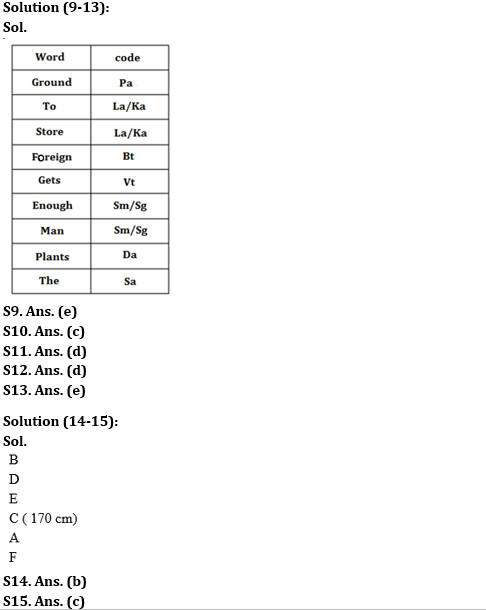Latest Banking jobs   »

# Reasoning Ability Quiz For RBI Grade B/ ECGC PO/ SIDBI Grade A Prelims 2022- 12th May

Directions (1-3): Study the following information carefully and answer the given questions.
Seven friends A, B, C, D, E, F and G have different height but not necessarily in the same order. Only two people are shorter than C. As many people are taller than C as shorter than G. B is taller than F but shorter than A. D is shorter than E. A is not the tallest person. F is not the shortest person. The height of third tallest person is 90 cm.

Q1. If the height of C is 69 cm then what is the possible height of B?
(a) 91 cm
(b) 82 cm
(c) 68 cm
(d) 65 cm
(e) 92cm

Q2. How many people are taller than F?
(a) Three
(b) Two
(c) Four
(d) Five
(e) None of these

Q3. Who among the following is just shorter than A?
(a) E
(b) D
(c) G
(d) B
(e) None of these

Directions (4-8): Study the following information carefully and answer the questions given below.
Seven people i.e. A, B, C, D, E, F and G live in nine storey building but not necessarily in the same order. In this building, ground floor is numbered as one, floor just above it is numbered as two and so on till the topmost floor is numbered as nine. Both vacant floors are not an even numbered floor. A lives on an even numbered floor and lives below 5th floor. There are two floors between A and F. Not more than one floor is between G and F but G does not live on an odd numbered floor. A does not live above G. There are two floors between G and both vacant floors. E lives below A. One person lives between E and C. B lives just above D.

Q4. How many people live between C and B?
(a) None
(b) Five
(c) Three
(d) Four
(e) None of these

Q5. Who among the following lives just above B?
(a) E
(b) F
(c) G
(d) H
(e) None

Q6. Who among the following lives on 6th floor?
(a) E
(b) F
(c) G
(d) H
(e) None of these

Q7. How many people live below C?
(a) None
(b) Five
(c) Three
(d) Four
(e) None of these

Q8. Who among the following lives on 7th floor?
(a) A
(b) B
(c) E
(d) D
(e) G

Directions (9-13): Study the following information carefully and answer the following question.
The following words are coded in this manner:
‘Ground to store’ is coded as ‘la ka pa’
‘Foreign ground gets’ is coded as ‘vt bt pa’
‘Enough man foreign plants’ is coded as ‘sm bt da sg’
‘Store to the plants’ is coded as ‘ka sa la da’

Q9. What is the code for ‘Store’?
(a) La
(b) Pa
(c) Ka
(d) Sa
(e) Cannot be determined

Q10. What is the code for ‘enough man to store’?
(a) pa la ka sg
(b) ka la vt da
(c) la ka sm sg
(d) da ka sm sg
(e) Cannot be determined

Q11. Which words are coded as ‘da vt’?
(a) Ground gets
(b) Foreign gets
(c) Foreign plants
(d) Plants gets
(e) Cannot be determined

Q12. If ‘Store can’ is coded as ‘ka dm’ then what is the code for ‘foreign to can’?
(a) ka sa ta
(b) Da bt dm
(c) Vt bt ka
(d) Bt la dm
(e) Cannot be determined

Q13. Which words are coded as ‘da vt sg’?
(a) Plants man gets
(b) Gets plants enough
(c) Enough plants Fareign
(d) Foreign plantsgets
(e) Cannot be determined

Directions (14-15): Study the information given below and answer the questions based on it.
Six persons A, B, C, D, E and F are standing in a straight line according to their height. No two persons are of the same height. C is 170 cm tall. D is not the tallest person. F is shorter than A, who is shorter than C and D. Only two persons are taller than E, but neither of them is C.

Q14. If D is 174 cm tall, then what could be the height of E?
(a) 168cm
(b) 171cm
(c) 165cm
(d) 187cm
(e) 175cm

Q15. Who is the tallest person?
(a) F
(b) C
(c) B
(d) A
(e) None of these

Solutions